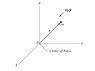# Torque and angular momentum with a central force

• fer Mnaj

#### fer Mnaj

Homework Statement
Consider the central force ##F (r) = - k / r^2 ˆr ## to which a particle of mass m is subject; where ˆr is the unit vector along the line connecting the center of forces with the position of the particle. Calculate the torque that F exerts on m and the angular momentum of the particle;
Calculate the torque that F exerts on m and the angular momentum of the particle;
both quantities with respect to O
Relevant Equations
τ= r x F L=rxp
HI

τ= r ˆr x - ##k / r ^ 2## ˆr= 0 right? since ˆr x ˆr is zero

#### Attachments

•Central force.png
2.2 KB · Views: 78